Home > PC > Chapter 11 > Lesson 11.1.2 > Problem11-28

11-28.
1. Example: Evaluate without the aid of a calculator: e3 ln 7. Homework Help ✎
Solution: e3 ln 7 = (eln 7)3 = 73 = 343.

Use this idea to solve the following problems.

1. e3 ln x

2. ln e5x

3. log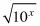4. 10(3+log x)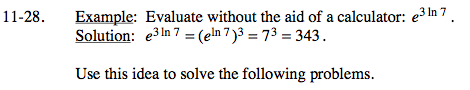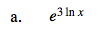$(\textit{e}^{\ln{\textit{x}}})^3$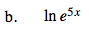$5\textit{x}\ln{\textit{e}}$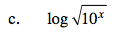$\log(10^x)^{1/2}$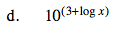$10^3 10^{\log{\textit{x}}}$